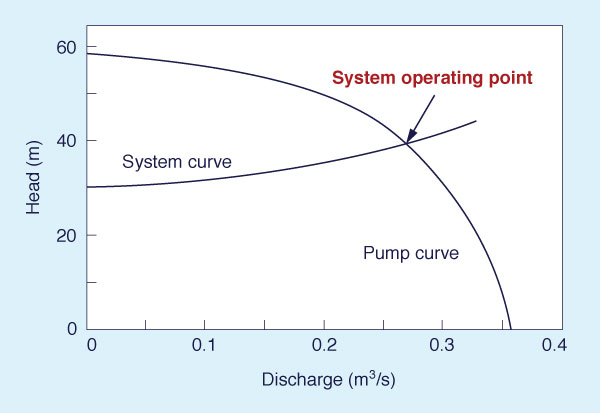online_pump_pipe_system:  Operating discharge and head in a pump-pipe systemDefinition sketch of a pump-pipe system

This online script calculates the operating discharge and head for a given hydraulic pump-system combination. The following hydraulic variables are specified as input:

• Pipe diameter D

• Pipe length L

• Friction factor f

• Upstream reservoir elevation E1

• Downstream reservoir elevation E2

• Entrance loss coefficient Ke

• Exit loss coefficient KE

• Sum of bend loss coefficient(s) [one or more bends] Kb

The script uses these values to calculate the values of a and b in the system curve   H = a + bQ2

The pump head-discharge relation is specified in one of two ways:

1. Theoretical:  An equation of the form  H = c - dQ2, where c and d are specified as input; or

2. Practical:  The number of values N in the arrays of pump head H and discharge Q relation (pump curve), followed by the array of H and Q.

Please note that the Q array (the independent variable) should increase monotonically, from small to large.

The script calculates the intersection of the two curves in the H-Q plane, and reports the corresponding Q and H as operating discharge and head.Thank you for running online_pump_pipe_system.   [160608 22:45]